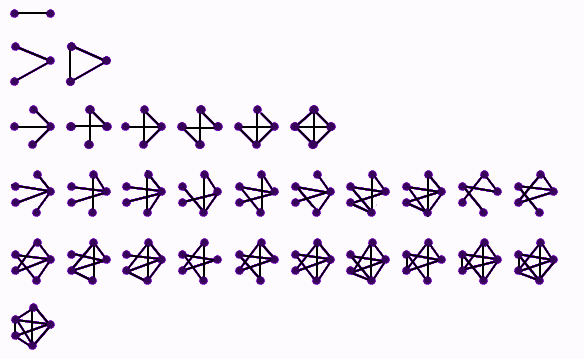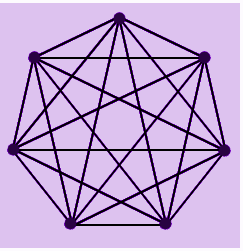# Connectivity

In Mathematics, the meaning of connectivity is one of the fundamental concepts of graph theory. It demands a minimum number of elements (nodes or edges) that require to be removed to isolate the remaining nodes into separated subgraphs. It is closely related to the principles of network flow problems. The connectivity of a graph is an essential measure of its flexibility as a network.

The word “connectivity” may belong to several applications in day to day life. Usually, it is referred to as the connection between two or more things or properties. In terms of different subjects, the definition of connectivity is described below:

1. In topology, the connected space is the topological space, which we cannot write in the form of union of two or more open non-empty subsets.
2. In the field of information and technology, the connectivity may relate to internet connectivity by which several individual computers, cell phones and LANs are connected to the global Internet.
3. In the field of transport planning, connectivity is also called permeability which relates to the limit to which urban structures limit the movement of vehicles and people.
4. The concept of pixel connectivity is used in the field of image processing.
5. Most importantly, in Mathematics, the term connectivity is utilized in graph theory. Graph connectivity is applicable in routing, network, network tolerance, transportation network, etc.

## Connectivity Definition

Connectivity is one of the essential concepts in graph theory. A graph may be related to either connected or disconnected in terms of topological space. If there exists a path from one point in a graph to another point in the same graph, then it is called a connected graph. Else, it is called a disconnected graph. Below are the diagrams which show various types of connectivity in the graphs.### Connectivity in Graph Theory

A graph is a connected graph if, for each pair of vertices, there exists at least one single path which joins them. A connected graph may demand a minimum number of edges or vertices which are required to be removed to separate the other vertices from one another. The graph connectivity is the measure of the robustness of the graph as a network.

In a connected graph, if any of the vertices are removed, the graph gets disconnected. Then the graph is called a vertex-connected graph. On the other hand, when an edge is removed, the graph becomes disconnected. It is known as an edge-connected graph.

### Properties of Connectivity

• The connected graph is called an undirected graph, which has at least one path between each pair of vertices.
• The graph that is connected by three vertices is called 1-vertex connected graph since the removal of any of the vertices will lead to disconnection of the graph.
• If in a connected graph, the removal of one edge leads to the disconnection of the graph, such a graph is called 1-edge connected graph.
• If there exists a set (say S) of edges (or vertices) in a connected graph, such that by removing all the edges of set S will result in a disconnected graph. Then the set S is called a cut set. If S consists of vertices, then it is called a vertex-cut set. Similarly, if it has edges, then it is called an edge-cut set.
• A bi-connected graph is a connected graph which has two vertices for which there are two disjoint paths between these two vertices.

## Connected Graphs in Discrete Maths

There are different types of connected graphs explained in Maths. They are:

• Fully Connected Graph
• K-connected Graph
• Strongly Connected Graph

Let us learn them one by one.

## Fully Connected Graph

In graph theory, the concept of a fully-connected graph is crucial. It is also termed as a complete graph. It is a connected graph where a unique edge connects each pair of vertices. In other words, for every two vertices of a whole or a fully connected graph, there is a distinct edge.

A fully connected graph is denoted by the symbol Kn, named after the great mathematician Kazimierz Kuratowski due to his contribution to graph theory. A complete graph Kn possesses n/2(n−1) number of edges. Given below is a fully-connected or a complete graph containing 7 edges and is denoted by K7.## K connected Graph

A graph is called a k-connected graph if it has the smallest set of k-vertices in such a way that if the set is removed, then the graph gets disconnected. Complete or fully-connected graphs do not come under this category because they don’t get disconnected by removing any vertices. A set of graphs has a large number of k vertices based on which the graph is called k-vertex connected. It could be one-connected, two-connected or bi-connected, three-connected or tri-connected.

## Strongly Connected Graph

A graph can be defined as a strongly connected graph if its every vertex can be reached from every other vertex in the graph. In other words, any directed graph is called strongly connected if there exists a path in each possible direction between each pair of vertices in the graph.

In a graph (say G) which may not be strongly connected itself, there may be a pair of vertices say (a and b) that are called strongly connected to each other if in case there exists a path in all the possible directions between a and b.

### Examples of Connectivity

Q.1: If a complete graph has a total of 20 vertices, then find the number of edges it may contain.

Solution: The formula for the total number of edges in a k15 graph is given by;

Number of edges = n(n-1)/2

= 20(20-1)/2

=10(19)

=190

Hence, it contains 190 edges.

Q.2: If a graph has 40 edges, then how many vertices does it have?

Solution: As we know,

Number of edges = n (n-1)/2

40 = n(n-1)/2

n(n-1) = 80

n2 – n – 80 = 0

On solving the above quadratic equation, we get;

n ≈ 9.45, -8.45

Since, the number of vertices cannot be negative.

Therefore, n ≈ 9